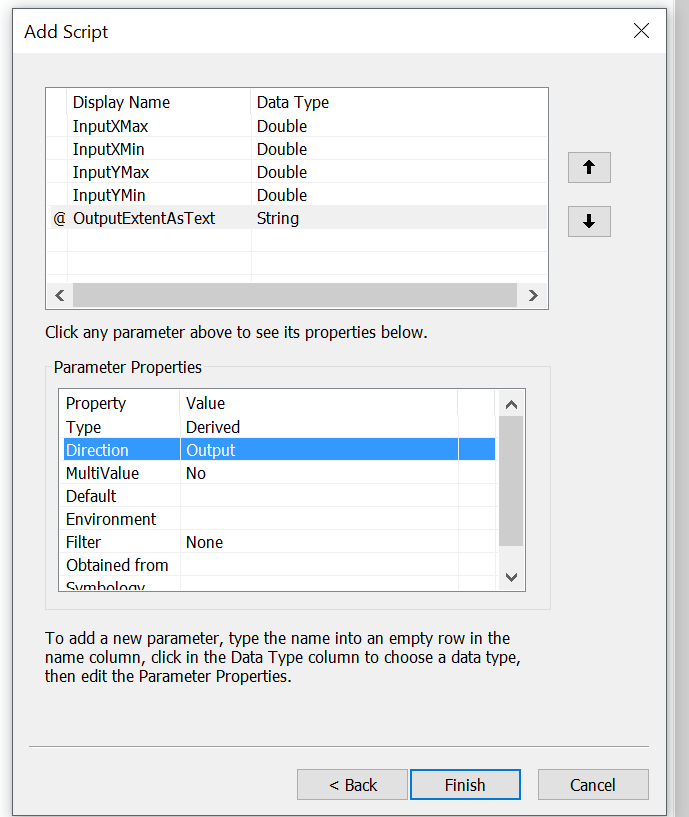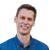# The order of the GeoprocessingParameters.Inputs is jumbled. Since my ArcPy script expects parameters in a specified order (i.e. xMin = arcpy.GetParameterAsText(0)) the model fails. Is this the intended behavior?

1507
6
12-27-2016 11:49 AMNew Contributor II

Sample code:

GeoprocessingParameters parameters = new GeoprocessingParameters(GeoprocessingExecutionType.AsynchronousSubmit);

etc

Output viewed in Fiddler:

Include_Attributes=false&Layout=S_Portrait_8x11&LineGraphics=&Map_Scale=6000&Map_Title=test2&PointGraphics=&PolyGraphics=&Spatial_Reference=2276&TimeStart=&Visiblelayers=&env%3AoutSR=%7B%22wkid%22%3A102100%2C%22latestWkid%22%3A3857%7D&f=json&returnM=false&returnZ=false&xMax=2480009&xMin=2476167&yMax=7106436&yMin=7103763

1 Solution

Accepted SolutionsRegular Contributor II

The parameters are actually handled through C++ (Map) and we surface that to the API as a IDictionary. It is true that it doesn't ensure that the order is preserved but is there any specific reason why we should have forced the ordering for the parameters? I give you that if you look the json which is send might be a bit confusing if you don't look the parameter names but not sure if there are other reasons that I have missed. In general you shouldn't really be looking the json for anything else than debugging purposes.

When parameters are send to the service the parameter order shouldn't matter since they are accessed through the arcPy on the order they are defined in the geoprocessing task that is published to the server. You need to make sure that your geoprocessing task and script has the same order for the parameters. From my testing it doesn't matter if we send the parameters in different order as long the tool and the script is correct.

For example

I use following simple script and create a tool in ArcMap using the same order of parameters while creating it.

``````import arcpy

def get_param(name, index):
param = arcpy.GetParameter(index)
arcpy.AddMessage("Input: " + name + " = " + str(param))
return param

def set_param(name, message, index, param):
arcpy.SetParameter(index, param)
arcpy.AddMessage("Output: " + name + " = " + str(param) + " Operation: " + message)

i = 0;
xMax_ = get_param("InputXMax", i)
i += 1
yMax_ = get_param("InputYMax", i)
i += 1
xMin_ = get_param("InputXMin", i)
i += 1
yMin_ = get_param("InputYMin", i)

i += 1
outputParam_ = "YMin = " + str(yMin_) +  ", XMin = " + str(xMin_) + ", YMax = " + str(yMax_) + ", XMax = " + str(xMax_) + "."
set_param("OutputExtentAsText", "Extent as a string", i, outputParam_)
‍‍‍‍‍‍‍‍‍‍‍‍‍‍‍‍‍‍‍‍‍‍‍‍‍‍‍‍‍‍‍‍‍‍‍‍‍‍‍‍‍‍‍‍‍‍‍‍‍‍‍‍‍‍‍‍‍‍‍‍‍‍‍‍‍‍‍‍‍‍‍‍‍‍‍‍‍‍‍‍‍‍‍‍‍‍‍‍‍‍‍‍‍‍‍‍‍‍‍‍‍‍‍‍‍‍‍‍‍‍‍‍‍‍‍‍‍‍‍``````

Then I publish it as a geoprocessing task to the ArcGIS Server (using 10.41 here). At this point I verified that the JSON definition for the task is correct and the parameters are defined in the same order.

I will execute the task using following code

``````var url = @"http://xxxxxxxx/arcgis/rest/services/OrderTest/GPServer/Script";

var parameters = new GeoprocessingParameters(GeoprocessingExecutionType.AsynchronousSubmit);

var results = await job.GetResultAsync();

{
Debug.WriteLine(log.Message);
}

Debug.WriteLine("The result is following : ");
var outputParameterValue = (results.Outputs.First().Value as GeoprocessingString).Value;
Debug.WriteLine(outputParameterValue);‍‍‍‍‍‍‍‍‍‍‍‍‍‍‍‍‍‍‍‍‍‍‍‍‍‍‍‍‍‍‍‍‍‍‍‍‍‍‍‍‍‍‍‍‍‍‍‍‍‍‍‍‍‍‍‍‍‍‍‍‍‍‍‍‍‍‍‍‍‍‍‍‍‍‍‍‍‍‍‍‍‍‍‍‍‍‍‍‍‍‍‍‍‍‍‍‍‍‍‍‍‍‍‍‍``````

I get following response which is expected

``````Sending request get result.
Sending request get geoprocessing service info.
Received response for get geoprocessing service info.
Job succeeded.
The result is following :
YMin = 4.0, XMin = 3.0, YMax = 2.0, XMax = 1.0.‍‍‍‍‍‍‍‍‍‍‍‍‍‍‍‍‍‍‍‍‍‍‍‍‍‍‍‍‍‍‍‍‍‍‍``````

If I change the values in the Inputs collection to another way around

``````parameters.Inputs.Add("InputYMin", new GeoprocessingDouble(4));

I get same results as expected

``````Sending request get result.
Sending request get geoprocessing service info.
Received response for get geoprocessing service info.
Job succeeded.
The result is following :
YMin = 4.0, XMin = 3.0, YMax = 2.0, XMax = 1.0.‍‍‍‍‍‍‍‍‍‍‍‍‍‍‍‍‍‍‍‍‍‍‍‍‍‍‍‍‍‍‍‍‍‍‍``````

If the parameters are not defined in the same order in the geoprocessing task (published to the server) that the script is expecting, the script output might be different that you might expect.

I created a new geoprocessing tool in ArcMap from the same script but this time I created parameters in different order. Note that I have changed the order so that X values are together and Y values are together contrast to Max values being together and Min values being together.I run the test with first set of parameters and will get 'wrong' results because the parameters are taken from wrong indexes.

``````Received response for get result.
Sending request get geoprocessing service info.
Received response for get geoprocessing service info.
Job succeeded.
The result is following :
YMin = 4.0, XMin = 2.0, YMax = 3.0, XMax = 1.0.‍‍‍‍‍‍‍‍‍‍‍‍‍‍‍‍‍‍‍‍‍‍‍‍‍‍‍‍‍‍``````

To fix the script I should change the indexes to correspond the order defined in the geoprocessing task.

Edit: Tried to make it more clear where I'm meaning geoprocessing task, which is published to the server, and GeoprocessingTask which is an API class.

6 RepliesRegular Contributor II

Can you share Geoprocessing tasks definition (parameters etc..) and can you verify that the order you get the parameters in the script is / isn't the same?New Contributor II

My original post shows the order of the parameters as coded and as captured in Fiddler. The list captured with Fiddler is clearly out of order. For example "xMin" was the first item added but it appears third from the end in Fiddler.

The GeoprocessingParameters Inputs are defined as IDictionary. The order of elements in IDictionay are not guaranteed since it is a hash.

Per Microsoft: "The IDictionary<TKey, TValue> interface allows the contained keys and values to be enumerated, but it does not imply any particular sort order"

I think ESRI should have used an OrderedDictionary to maintain the order of the InputsRegular Contributor II

The parameters are actually handled through C++ (Map) and we surface that to the API as a IDictionary. It is true that it doesn't ensure that the order is preserved but is there any specific reason why we should have forced the ordering for the parameters? I give you that if you look the json which is send might be a bit confusing if you don't look the parameter names but not sure if there are other reasons that I have missed. In general you shouldn't really be looking the json for anything else than debugging purposes.

When parameters are send to the service the parameter order shouldn't matter since they are accessed through the arcPy on the order they are defined in the geoprocessing task that is published to the server. You need to make sure that your geoprocessing task and script has the same order for the parameters. From my testing it doesn't matter if we send the parameters in different order as long the tool and the script is correct.

For example

I use following simple script and create a tool in ArcMap using the same order of parameters while creating it.

``````import arcpy

def get_param(name, index):
param = arcpy.GetParameter(index)
arcpy.AddMessage("Input: " + name + " = " + str(param))
return param

def set_param(name, message, index, param):
arcpy.SetParameter(index, param)
arcpy.AddMessage("Output: " + name + " = " + str(param) + " Operation: " + message)

i = 0;
xMax_ = get_param("InputXMax", i)
i += 1
yMax_ = get_param("InputYMax", i)
i += 1
xMin_ = get_param("InputXMin", i)
i += 1
yMin_ = get_param("InputYMin", i)

i += 1
outputParam_ = "YMin = " + str(yMin_) +  ", XMin = " + str(xMin_) + ", YMax = " + str(yMax_) + ", XMax = " + str(xMax_) + "."
set_param("OutputExtentAsText", "Extent as a string", i, outputParam_)
‍‍‍‍‍‍‍‍‍‍‍‍‍‍‍‍‍‍‍‍‍‍‍‍‍‍‍‍‍‍‍‍‍‍‍‍‍‍‍‍‍‍‍‍‍‍‍‍‍‍‍‍‍‍‍‍‍‍‍‍‍‍‍‍‍‍‍‍‍‍‍‍‍‍‍‍‍‍‍‍‍‍‍‍‍‍‍‍‍‍‍‍‍‍‍‍‍‍‍‍‍‍‍‍‍‍‍‍‍‍‍‍‍‍‍‍‍‍‍``````

Then I publish it as a geoprocessing task to the ArcGIS Server (using 10.41 here). At this point I verified that the JSON definition for the task is correct and the parameters are defined in the same order.

I will execute the task using following code

``````var url = @"http://xxxxxxxx/arcgis/rest/services/OrderTest/GPServer/Script";

var parameters = new GeoprocessingParameters(GeoprocessingExecutionType.AsynchronousSubmit);

var results = await job.GetResultAsync();

{
Debug.WriteLine(log.Message);
}

Debug.WriteLine("The result is following : ");
var outputParameterValue = (results.Outputs.First().Value as GeoprocessingString).Value;
Debug.WriteLine(outputParameterValue);‍‍‍‍‍‍‍‍‍‍‍‍‍‍‍‍‍‍‍‍‍‍‍‍‍‍‍‍‍‍‍‍‍‍‍‍‍‍‍‍‍‍‍‍‍‍‍‍‍‍‍‍‍‍‍‍‍‍‍‍‍‍‍‍‍‍‍‍‍‍‍‍‍‍‍‍‍‍‍‍‍‍‍‍‍‍‍‍‍‍‍‍‍‍‍‍‍‍‍‍‍‍‍‍‍``````

I get following response which is expected

``````Sending request get result.
Sending request get geoprocessing service info.
Received response for get geoprocessing service info.
Job succeeded.
The result is following :
YMin = 4.0, XMin = 3.0, YMax = 2.0, XMax = 1.0.‍‍‍‍‍‍‍‍‍‍‍‍‍‍‍‍‍‍‍‍‍‍‍‍‍‍‍‍‍‍‍‍‍‍‍``````

If I change the values in the Inputs collection to another way around

``````parameters.Inputs.Add("InputYMin", new GeoprocessingDouble(4));

I get same results as expected

``````Sending request get result.
Sending request get geoprocessing service info.
Received response for get geoprocessing service info.
Job succeeded.
The result is following :
YMin = 4.0, XMin = 3.0, YMax = 2.0, XMax = 1.0.‍‍‍‍‍‍‍‍‍‍‍‍‍‍‍‍‍‍‍‍‍‍‍‍‍‍‍‍‍‍‍‍‍‍‍``````

If the parameters are not defined in the same order in the geoprocessing task (published to the server) that the script is expecting, the script output might be different that you might expect.

I created a new geoprocessing tool in ArcMap from the same script but this time I created parameters in different order. Note that I have changed the order so that X values are together and Y values are together contrast to Max values being together and Min values being together.I run the test with first set of parameters and will get 'wrong' results because the parameters are taken from wrong indexes.

``````Received response for get result.
Sending request get geoprocessing service info.
Received response for get geoprocessing service info.
Job succeeded.
The result is following :
YMin = 4.0, XMin = 2.0, YMax = 3.0, XMax = 1.0.‍‍‍‍‍‍‍‍‍‍‍‍‍‍‍‍‍‍‍‍‍‍‍‍‍‍‍‍‍‍``````

To fix the script I should change the indexes to correspond the order defined in the geoprocessing task.

Edit: Tried to make it more clear where I'm meaning geoprocessing task, which is published to the server, and GeoprocessingTask which is an API class.byEsri Frequent Contributor

The order of query parameters should not ever matter in web requests, and we can't guarantee a specific order of those parameters.

If this is causing an issue, it's either a GP or Server problem. I don't really know ArcPy, but from the looks of it, your script is using parameter indexes rather than parameter names to get the input values. ie arcpy.GetParameterAsText(0). That's most likely the problem.

Try the GetParameterInfo first to figure out which index a certain parameter is at.New Contributor II

As Morten and Antti mentioned the order of the GeoProcessing paramters does not matter. The order is defined when you publish the service. The arcpy script may access them by index.

The Silverlight API happened to keep them in order. When inspecting the web request with Fiddler the only thing I noticed between the working Silverlight application and the failing RT application was the order of the parameters. After further inspection I realized my existing service was set up for Synchronous execution. I created a duplicate service configured for Asynchronous execution. Problem solved.

Thank you Antti for all your work on the problem. I appreciate your help. I am embarrassed to say I have spent days on this. It just confirms my life philosophy: Don't sweat the big stuff, it's the little things that will bite you in the ass.Regular Contributor II

Glad that you get it working. We are going to make using GP a bit easier in next release where you can create parameters using a factory method and it takes care of using correct execution type and creates all the parameters for you.